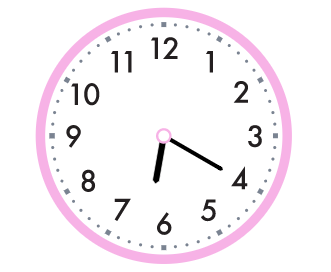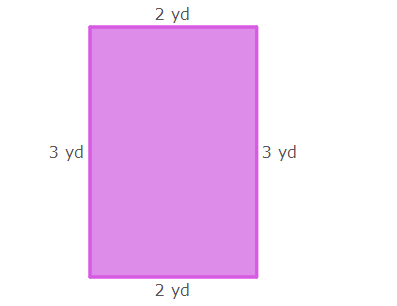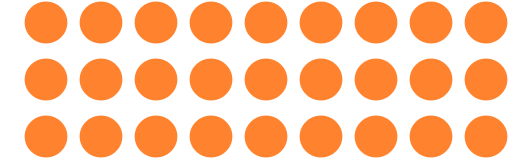Area using standard Units
100

What a multiplication fact for the following picture:3 x 5 = 15, 5 x 3 = 15

100

10 Divided By 5

2
100

What is the missing side length of a rectangle with an area of 48 sq. inches and a width of 8 inches.

6 inches

100

What is the area of a squares with a side measurement of 6 cm?

36 sq cm

200

Write a multiplication equation for the array:5x2=10 or 2 x 5=10

200

Round 54 to the nearest ten

50

200

Why would you need to calculate area to put a rug in a room?

Area measures how much space something takes up. You need to calculate area to make sure the rug will fit!

200

What is the area a rectangle length of 7 inches and a width of 9 inches?

63 square inches

300

What is 100x8

800

300

What time is shown?6:20

300

What is the perimeter of this rectangle?10 yards (2+3+2+3)

300

What is the area of square with a length of 5 ft?

25 square feet

400

Mr. Hughes completed 8 chores each day for an entire week. How many chores did he complete by the end of the week?

8x7=56

400

Round 658 to the nearest hundred

700

400

What is the area of this rectangle?6 square yards (2x3)

400

Draw a shape that has an area of 16 square units.

500

Draw an array to solve

3x9500

Why can't you divide 0 by 0?

Because you can't split up nothing into zero groups. It doesn't make sense!

500

Fritz needs a play pen that has a perimeter of area of 36 square feet. Mr. Hughes builds a rectangular pen that 7 feet wide and 5 feet long. Is the pen big enough?

No because 7 x 5 = 35! It's one square foot short.

500

Draw a shape that is 8 units long and 6 units wide. What is the area?

48 square units.

Click to zoom